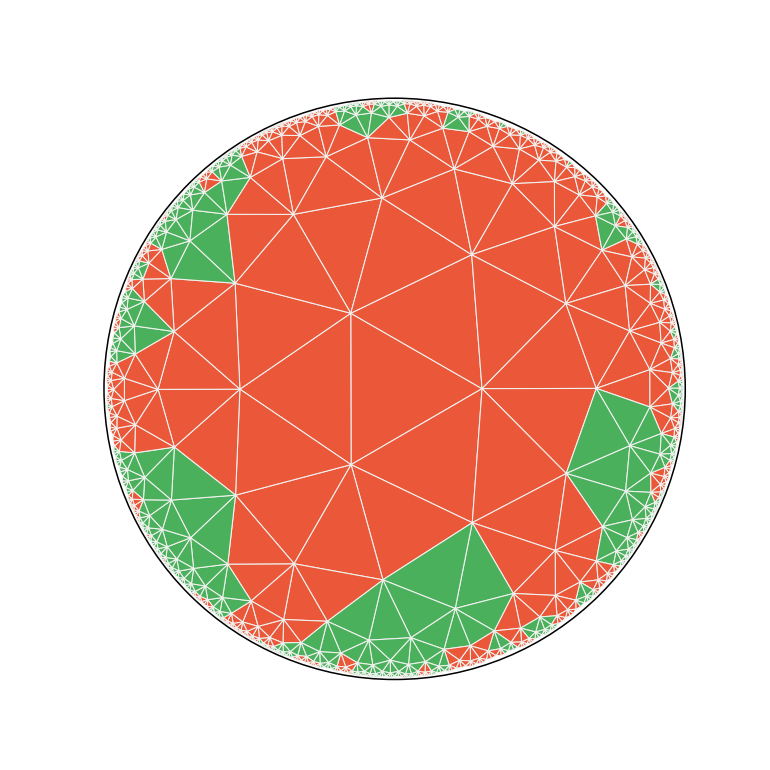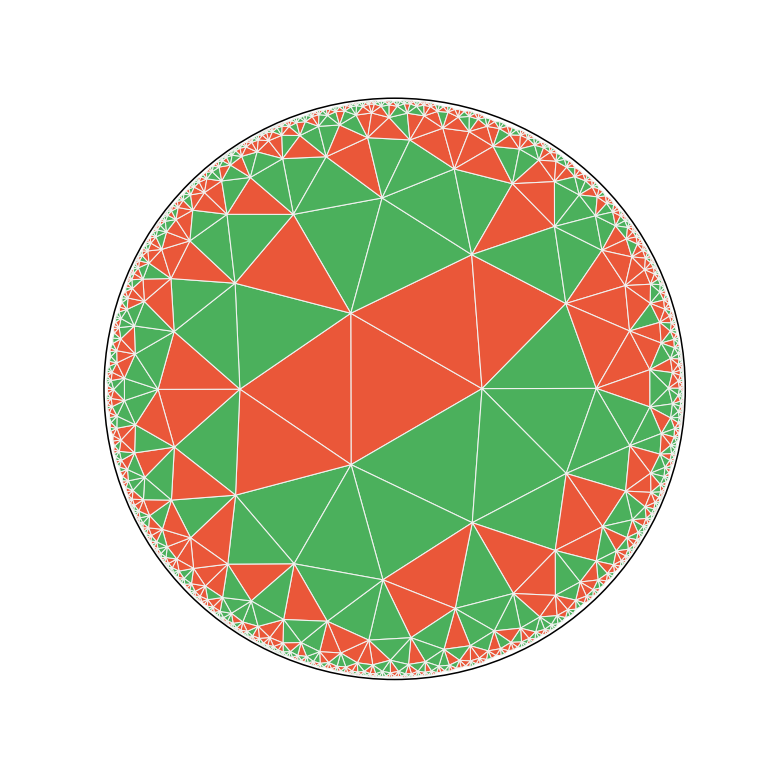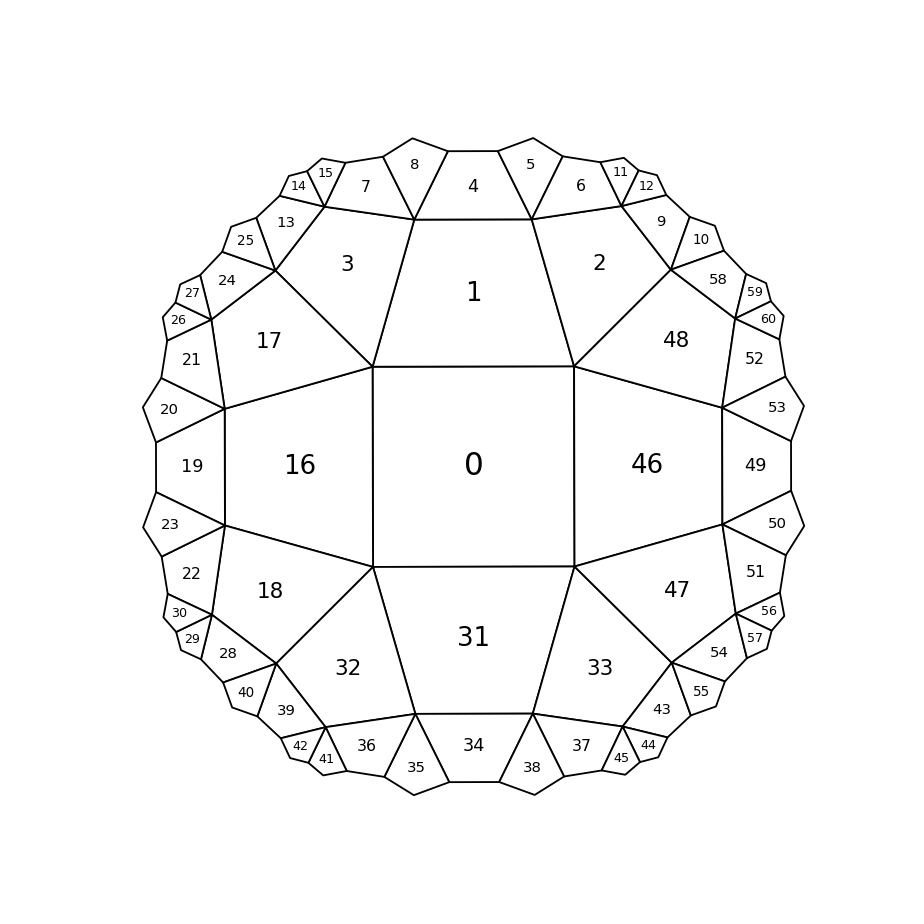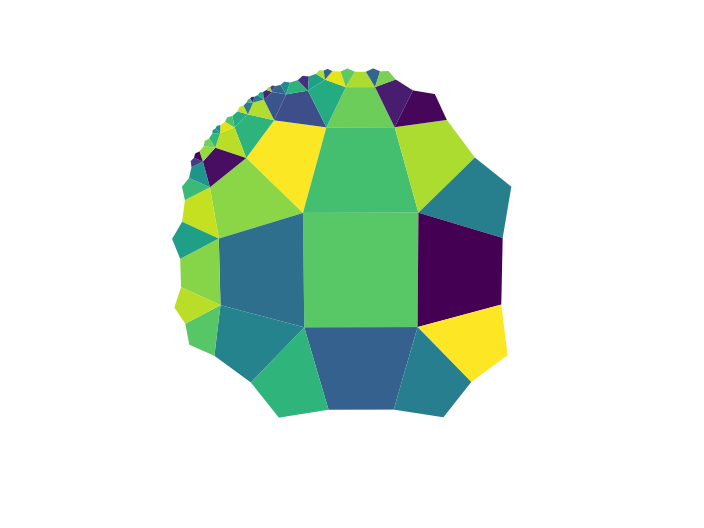# Hyperanimator

hypertiling offers a simple API to animate hyperbolic tilings using the FuncAnimation feature of matplotlib. The hyperanimator class is a wrapper which simplifies the associated syntax and let’s you realize simple animation with very little effort

:

import numpy as np
from matplotlib import pyplot as plt
import matplotlib.cm as cmap

:

from hypertiling import HyperbolicTiling
from hypertiling.graphics.plot import convert_polygons_to_patches


enable interactive plot in notebook

:

%matplotlib notebook


define a function which takes one configuration to the next. In this case we implement a simple Ising model

:

J = 1 # coupling constant (positiv: ferromagnet, negativ: antiferromagnet)

def step(state, beta):

for i in range(len(state)):

site = np.random.choice(len(state))

nbrhood = 0
for n in nbrs[site]:
nbrhood += state[n]

phi_old = state[site]
phi_new = -state[site]
E1 = -J*phi_old*nbrhood
E2 = -J*phi_new*nbrhood
dE = E2-E1

if dE < 0:
state[site] = phi_new
elif np.random.rand() < np.exp(-beta*dE):
state[site] = phi_new

return state


construct the tiling and generate an initial state for the model

:

T = HyperbolicTiling(3, 7, 6)
nbrs = T.get_nbrs_list()

colors = 2*np.random.choice(2, len(T))-1


## Live Animation

:

# import hyperanimator class
from hypertiling.graphics.animation import AnimatorLive

:

# create figure
fig, axs = plt.subplots(1,1,figsize=(7,7))

# plot tiling using built in function "poly2patch"
patchkwargs = {"cmap": cmap.RdYlGn, "edgecolor": "#f1f2f0ff", "lw": 0.7, "clim":[-1.5,1.5]}
pgons = convert_polygons_to_patches(T, colors, **patchkwargs)

# ------- this is the animation part -------
# note: the argument "frames" is only relevant for saving the animation, in the
# live plot it will run forever (i.e. until manually stopped)

# extra arguments to the step functions; leave away if there are none
stepargs = {"beta": 2}
# arguments to be passed through to the matplotlib FuncAnimator
animargs = {"frames": 300, "interval": 5, "blit": True}
# the actual animator
ani = AnimatorLive(colors, fig, pgons, step, stepargs, animargs)
# -------

axs.set_xlim(-1.02,1.02)
axs.set_ylim(-1.02,1.02)
axs.axis("off"); axs.set_aspect('equal'); plt.show()hyperanimations can be easily saved like this

:

ani.save("/home/user/anim.mp4", fps=10, codec="libx264")


## List Animation

:

from hypertiling.graphics.animation import AnimatorList


Pre-compute some data and store it in 2D array-like container. Here we use our Ising model, but of course data can come from anywhere

:

# first dimension: max number of frames, second dimension: len(tiling)
colors_list = np.zeros((100,len(T)))

for i in range(100):
colors_list[i] = step(colors, beta=0.1)

:

# create figure
fig, axs = plt.subplots(1,1,figsize=(7,7))

# plot tiling using built in function "poly2patch"
patchkwargs = {"cmap": cmap.RdYlGn, "edgecolor": "#f1f2f0ff", "lw": 0.7, "clim":[-1.5,1.5]}
pgons = convert_polygons_to_patches(T, colors, **patchkwargs)

# ------- this is the animation part -------

# arguments to be passed through to the matplotlib FuncAnimator
animargs = {"interval": 200, "blit": True}

# the actual animator
ani = AnimatorList(colors_list, fig, pgons, animargs)

# -------

axs.set_xlim(-1.02,1.02)
axs.set_ylim(-1.02,1.02)
axs.axis("off"); axs.set_aspect('equal'); plt.show()[ ]:

ani.save("/home/user/anim.mp4", fps=10, codec="libx264")


## Path Animation

With AnimatorPath you can parse a predefined path which the camera will track throughout the animation. Here we will demonstrate how walking in a circle on a hyperbolic disk we accumulate rotation.

:

from hypertiling.graphics.plot import plot_tiling
from hypertiling.graphics.animation import AnimatorPath

:

T = HyperbolicTiling(4, 5, 3)
T.rotate(-np.pi/4)

:

plot_tiling(T)
for poly in T.polygons:
x, y = poly.centerP().real, poly.centerP().imag
plt.text(x, y, f"{poly.idx}", ha="center", va="center", fontsize=17*(1-0.7*np.abs(x*x+y*y)))Our path will be: 0 → 1 → 2 → 48 → 46 → 0

We paint the cells with random colors in order to make it easier to follow the movement

:

path = [0, 1, 2, 48, 46, 0]
colors = np.random.rand(len(T))

:

fig, ax = plt.subplots()
ani = AnimatorPath(colors, fig, ax, T, path)Note that while our path returns back to central polygon, the lattice is now rotated by $$\frac{\pi}{2}$$ in clock-wise direction. This is due to the curvature of the space and does not happen in flat space (try it out on a piece of grid paper yourself!).

[ ]:

ani.save("/home/user/anim.mp4", fps=60)